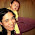## 26 June 2011

### An amazing chemical anagram

The names of 30 elements -
hydrogen + zirconium + tin + oxygen + rhenium + platinum +
tellurium + terbium + nobelium + chromium + iron + cobalt +
carbon + aluminum + ruthenium + silicon + ytterbium + hafnium +
sodium + selenium + cerium + manganese + osmium + uranium +
nickel + praseodymium + erbium + vanadium + thallium + plutonium
- can be anagrammed to form a different group of 30 elements:
nitrogen + zinc + rhodium + helium + argon + neptunium +
beryllium + bromine + lutetium + boron + calcium + thorium +
niobium + lanthanum + mercury + fluorine + bismuth + actinium +
silver + cesium + neodymium + magnesium + xenon + samarium +
scandium + europium + berkelium + palladium + antimony + thulium.
AND...  if you replace the elements with their atomic numbers -
1 + 40 + 50 + 8 + 75 + 78 +
52 + 65 + 102 + 24 + 26 + 27 +
6 + 13 + 44 + 14 + 70 + 72 +
11 + 34 + 58 + 25 + 76 + 92 +
28 + 59 + 68 + 23 + 81 + 94
=
7 + 30 + 45 + 2 + 18 + 93 +
4 + 35 + 71 + 5 + 20 + 90 +
41 + 57 + 80 + 9 + 83 + 89 +
47 + 55 + 60 + 12 + 54 + 62 +
21 + 63 + 97 + 46 + 51 + 69
[= 1416]
- the two groups are still equal.

From a group of "Anagrammy Winners" composed by Mike Keith.  (See his anagram of Poe's "The Raven.")

1.2.